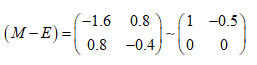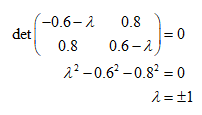# I. 2. Write the matrix for reflection in line OA

Thus line of reflection pass through the origin the transformation is linear and could be expressed by 2 x 2 matrix. 1. Reflect base vectors e1, e2. 2. Images e1' and e2' are columns of the matrix of linear transformation. 3. Check the representation by means of the picture "lion".

## Fixed points (construction step 6)

Method 1 (Experimental): Move with point B to get location, where B = B'. Discover all such position. Method 2 (Algebraic): Matrix equation of transformation X' = MX together with condition for fixed points X' = X give equation X = MX for unknown fixed point X. Rearrangement in homogenous form: could be solved by Gauss elimination. GeoGebra command ReducedRowEchelonForm(matrix) of the matrix (M - E) returns the equivalent echelon form. Matrix for reflection in line OA, O=(0,0), A =(1, 2) is M = {{-0.6, 0.8},{0.8, 0.6}}, thusUsing back-substitution, unknown coordinates x, y can be solved for. x - 0.5 y = 0, hence y = 2x.

## Eigenvectors = Fixed directions (construction step 7)

Method 1 (experimental): Move with dynamic points B, C and investigate the relationship of line BC and its image B'C'. We look for position where line BC is parallel with B'C'. Method 2 (algebraic): Fixed direction (=eigenvectors) X fulfils equation X' = λX for some λ, i.e. we should find coefficient λ for which λX = MX has nonzero solution X. Rearrangement in homogenous form (M-λE)X = o gives the necessary condition for nonzero solution:

det(M-λE) = 0Change the value of slider λ. Only two values induce singular matrix (M-λE).
• λ=1 gives direction vector of mirror line (M-E)X = o; x + 2y = 0, X1=(-2t, t)
• λ=-1 gives f normal vector of mirror line (M+E)X = o; x - 0.5y = 0, X2 = (t, 2t)